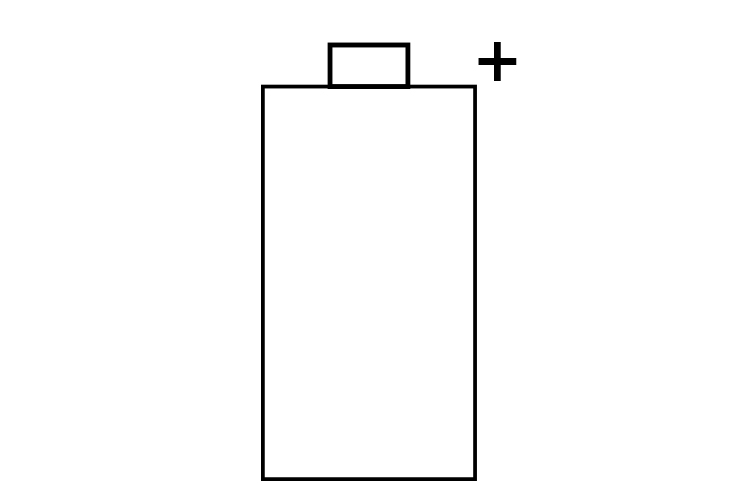# How to remember + or -

On a battery or diagram, which is the +ve and which is the -ve end?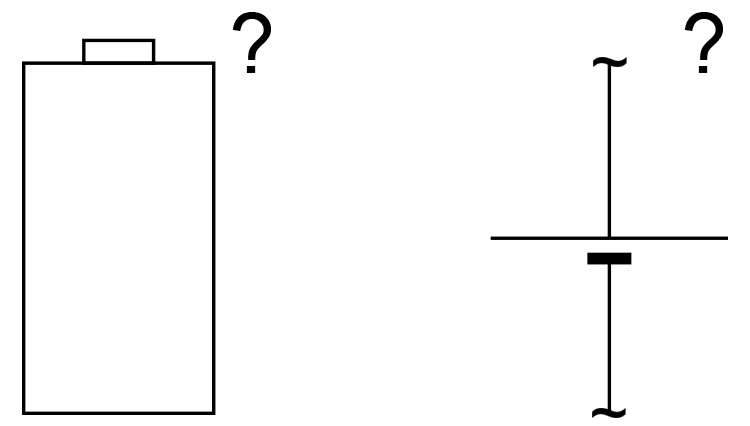To remember the +ve end, first decide whether a +ve symbol ( + ) or -ve symbol ( – ) has a longer line in total: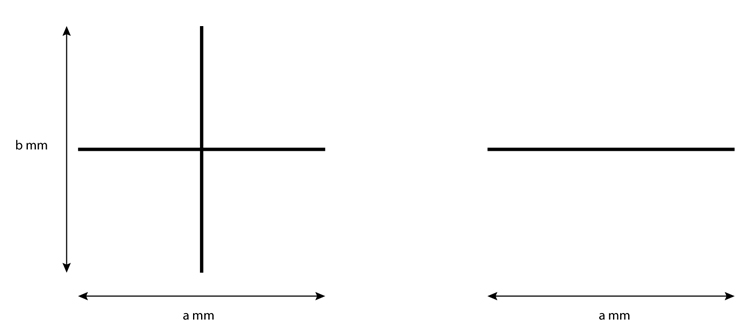As you can see the +ve needs a longer line in total, i.e. a+b\ \mm.

So, in the diagram below, which is the longer line: x\ \mm or y\ \mm?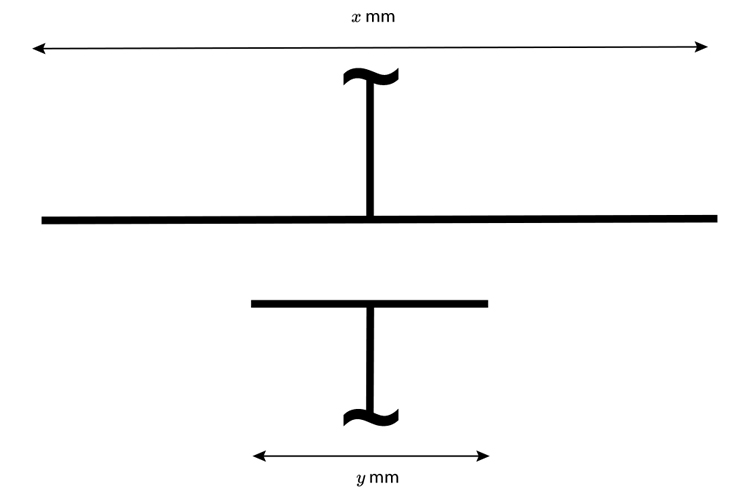Again, it's easy to see that x\ \mm is the longer line.

We match the two longer lines together, i.e. the +ve with the longer line. Hence the electrical diagram is marked: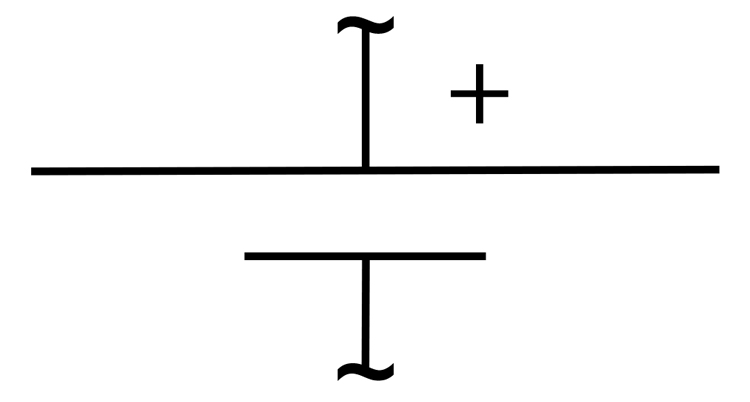Now, on a real battery, we can use the same idea.

So which is the longer line: a+b+c+d+e\ \mm or f\ \mm?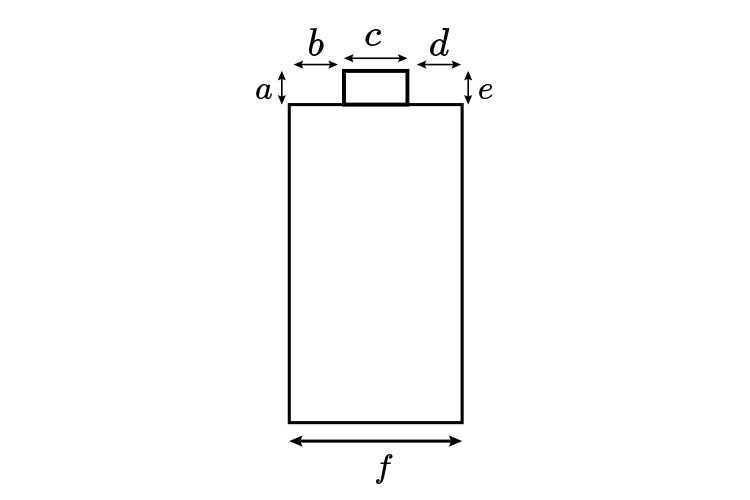Again, it's easy to see that a+b+c+d+e\ \mm is the longer.

So we match the two longer lines together, i.e. the +ve with the longer line. Hence the battery is marked: